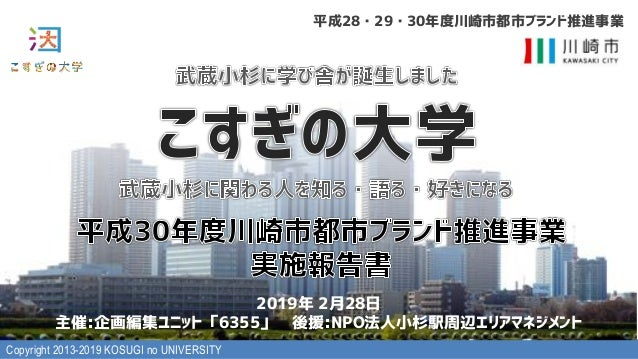Successfully reported this slideshow.Upcoming SlideShare
×

# 平成30年度川崎市都市ブランド推進事業実施報告書

5,938 views

Published on

Published in: Design
• Full Name
Comment goes here.

Are you sure you want to Yes No• Be the first to comment

• Be the first to like this

### 平成30年度川崎市都市ブランド推進事業実施報告書

1. 1. Copyright 2013-2019 KOSUGI no UNIVERSITY ) (( 35
2. 2. Copyright 2013-2019 KOSUGI no UNIVERSITY l  UW a a l  a B e c f l  l  B
3. 3. Copyright 2013-2019 KOSUGI no UNIVERSITY UW a a
4. 4. Copyright 2013-2019 KOSUGI no UNIVERSITY UW a a 3 P A O L UW a + 23+1 t n i t n L a a a
5. 5. Copyright 2013-2019 KOSUGI no UNIVERSITY 3 +) 2 i+) 2 + 0. d+) 2 + hd × w ) : ) - : +
6. 6. Copyright 2013-2019 KOSUGI no UNIVERSITY /2 l BPITT M m +) 1 2 -
7. 7. Copyright 2013-2019 KOSUGI no UNIVERSITY 0- l m +) 2 +
8. 8. Copyright 2013-2019 KOSUGI no UNIVERSITY )
9. 9. Copyright 2013-2019 KOSUGI no UNIVERSITY B C a a t W ea l  a a H a B H × 3 i × 3 +) × / %. 4 .. l  N H B s 87 - c UILM V 5F5B5 p / c / c 87 2 c B5 v + l  B -2. (
10. 10. Copyright 2013-2019 KOSUGI no UNIVERSITY B C a a t W ea l  a l × T n na B Hl m 3 + l  l n n a B + jc i ×jc h+ ×c P[[ 3(( T LM PI M VM[(SI[ P SW)1+ (]M -) 12+.0 l  × d Sw B y p c c l v m /
11. 11. Copyright 2013-2019 KOSUGI no UNIVERSITY B a l  a t a l a B H × × 3 l  a B /( i - l m 776 j 0 ++ t jl v m jc c × l m ×c P[[ 3((OWW OT( 21O c l  a90 B × × d c P[[ 3((SI[ P SW)1+ PI[MVI TWO KWU(MV[ (+) 2()+(+/(+ .+.0 0
12. 12. Copyright 2013-2019 KOSUGI no UNIVERSITY B a l  a t e a B H × × 3 l  UW a ( B c 0 c c s c l  B 3 + c c @ ! 9 s u . × + .
13. 13. Copyright 2013-2019 KOSUGI no UNIVERSITY B a l  a t e a B H × × 3 i o P[[ 3(( SIVITWKW ( I [ KTM( 1./.+ l v m h P[[ 3(( SIVITWKW ( I [ KTM( /1-)1 v i t P[[ 3(( [W VVM KW ( )+)-(+) 1( )( 2(-. .-/ P[ UT
14. 14. Copyright 2013-2019 KOSUGI no UNIVERSITY B E a l  a l  10- 85 B +) 1 ) B a b i × j l  2: 6: +) 1 0 B l +)-)m l I BIV[M vm j l  30 a +) 1 - 2 c w i l m j
15. 15. Copyright 2013-2019 KOSUGI no UNIVERSITY
16. 16. Copyright 2013-2019 KOSUGI no UNIVERSITY +1 i i n t p o j i i i s i i k t ui o j i ou i u ui r oo ×l/ ..m hP[[ 3(( SW O VW V ] KWU( 9IKM WWShP[[ 3(( NIKM WWS KWU(SW O VW V ]( o h hSI[ P SW)1+ 4OUI T KWU
17. 17. Copyright 2013-2019 KOSUGI no UNIVERSITY
18. 18. Copyright 2013-2019 KOSUGI no UNIVERSITY a h /- - h +/ g - 2 h s 87 .) h /. . h r + h // / 1 h AC5 g / / hUILM V 5F5B5 p +1 g / ) h - h /0 0 h +1 h /1 1 +. h +0 g /2 2 - h BPITT M - g 0) 2 + h 87 ++ h 0 ) +0 h v . . g ) h :85C A u l m h 0+ 2 h s + h 0 + - h t o +- g + . h B5 v +/ h 0- + h ++ h 0. + 1 h t EWT + . -2. g 3 i i × )